# What is Cryptography? — An Introduction to Cryptographic AlgorithmsEncryption is essentially important because it secures data and information from unauthorized access and thus maintains confidentiality. Here’s an article to help you understand “w_hat is cryptography “_ and how can it be used to protect corporate secrets, secure classified information, and personal information to guard against things like identity theft.

• Introduction to Cryptography?
• Encryption Algorithms
• How various Cryptographic Algorithms Works?

Now, I’m going to take help of an example or a scenario to explain what is cryptography?

Let’s say there’s a person named Andy. Now suppose Andy sends a message to his friend Sam who is on the other side of the world. Now obviously he wants this message to be private and nobody else should have access to the message. He uses a public forum, for example, WhatsApp for sending this message. The main goal is to secure this communication.Let’s say there is a smart guy called Eaves who secretly got access to your communication channel. Since this guy has access to your communication, he can do much more than just eavesdropping, for example, he can try to change the message. Now, this is just a small example. What if Eave gets access to your private information? The result could be catastrophic.

So how can Andy be sure that nobody in the middle could access the message sent to Sam? That’s where Encryption or Cryptography comes in. Let me tell you ” What is Cryptography “.

# What Is Cryptography?

Cryptography is the practice and study of techniques for securing communication and data in the presence of adversaries.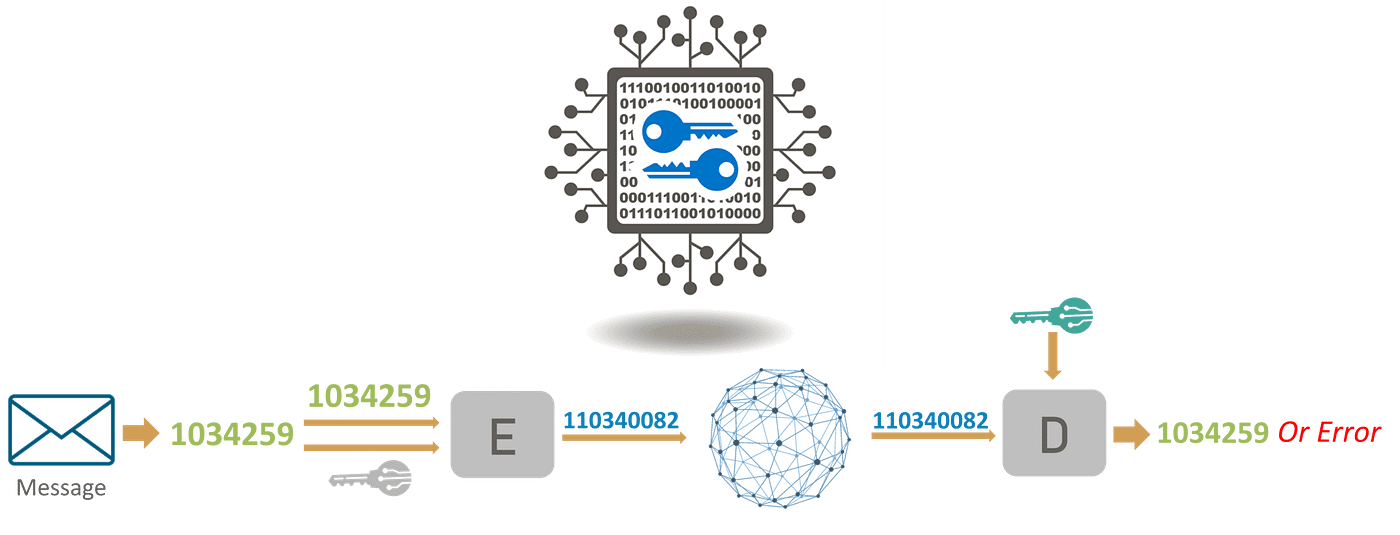Alright, now that you know ” what is cryptography ” let’s see how cryptography can help secure the connection between Andy and Sam.

So, to protect his message, Andy first converts his readable message to unreadable form. Here, he converts the message to some random numbers. After that, he uses a key to encrypt his message, in Cryptography, we call this ciphertext.

Andy sends this ciphertext or encrypted message over the communication channel, he won’t have to worry about somebody in the middle of discovering his private messages. Suppose, Eaves here discover the message and he somehow manages to alter it before it reaches Sam.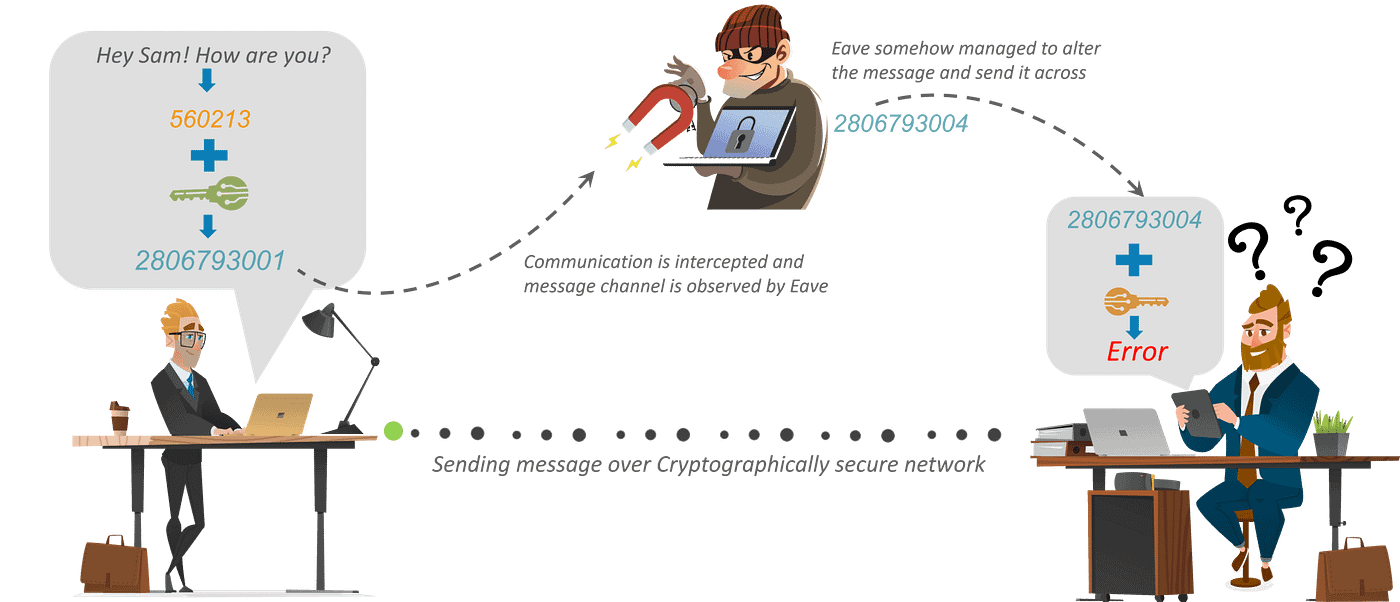Now, Sam would need a key to decrypt the message to recover the original plaintext. In order to convert the ciphertext into plain text, Sam would need to use the decryption key. Using the key he would convert the ciphertext or the numerical value to the corresponding plain text.

After using the key for decryption what will come out is the original plaintext message, is an error. Now, this error is very important. It is the way Sam knows that message sent by Andy is not the same as the message that he received. Thus, we can say that encryption is important to communicate or share information over the network.

# Encryption Algorithms

Cryptography is broadly classified into two categories: Symmetric key Cryptography and Asymmetric key Cryptography (popularly known as public key cryptography).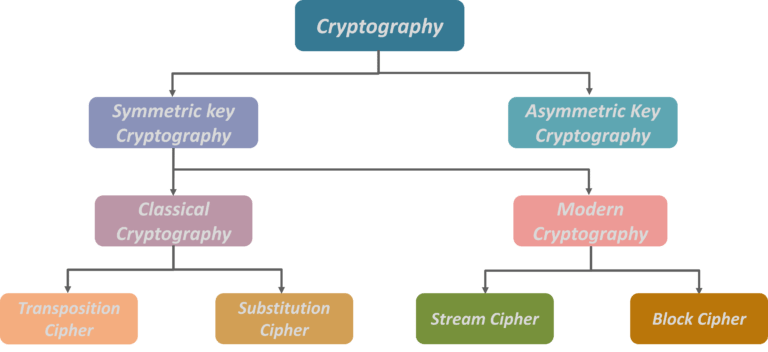Now Symmetric key Cryptography is further categorized as Classical Cryptography and Modern Cryptography.

Further drilling down, Classical Cryptography is divided into Transposition Cipher and Substitution Cipher. On the other hand, Modern Cryptography is divided into Stream Cipher and Block Cipher.

So, let’s understand these algorithms with examples.

# How various Cryptographic Algorithms Works?

## Symmetric Key Cryptography

An encryption system in which the sender and receiver of a message share a single, common key that is used to encrypt and decrypt the message. The most popular symmetrickey system is the Data Encryption Standard (DES).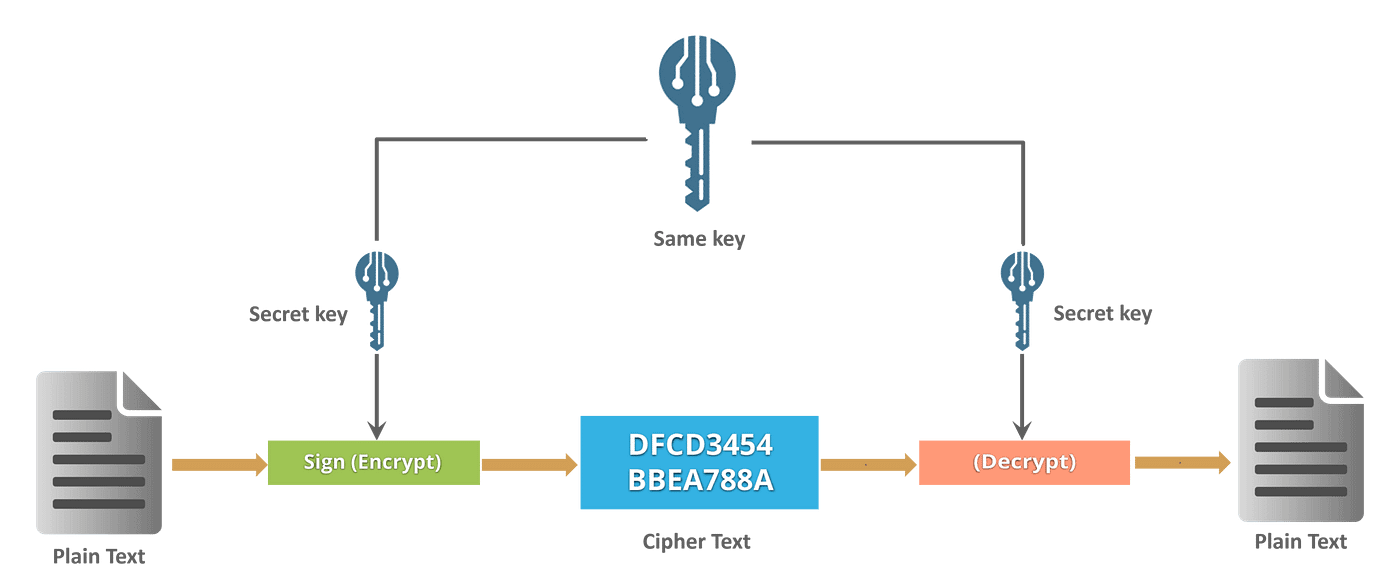## Transposition Ciphers

In Cryptography, a transposition cipher is a method of encryption by which the positions held by units of plaintext (which are commonly characters or groups of characters) are shifted according to a regular system, so that the ciphertext constitutes a permutation of the plaintext.

That is, the order of the units is changed (the plaintext is reordered). Mathematically, a bijective function is used on the characters’ positions to encrypt and an inverse function to decrypt.

Example: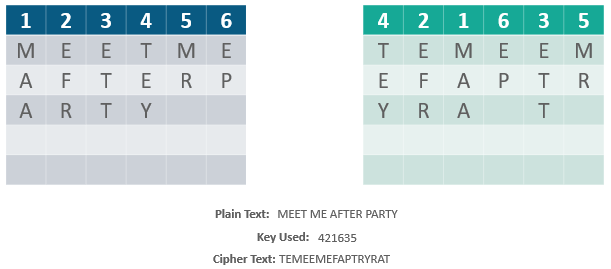## Substitution Cipher

Method of encryption by which units of plaintext are replaced with ciphertext, according to a fixed system; the “units” may be single letters (the most common), pairs of letters, triplets of letters, mixtures of the above, and so forth.

Example:

Consider this example shown on the slide: Using the system just discussed, the keyword “zebras” gives us the following alphabets:## Stream Cipher

Symmetric or secret-key encryption algorithm that encrypts a single bit at a time. With a Stream Cipher, the same plaintext bit or byte will encrypt to a different bit or byte every time it is encrypted.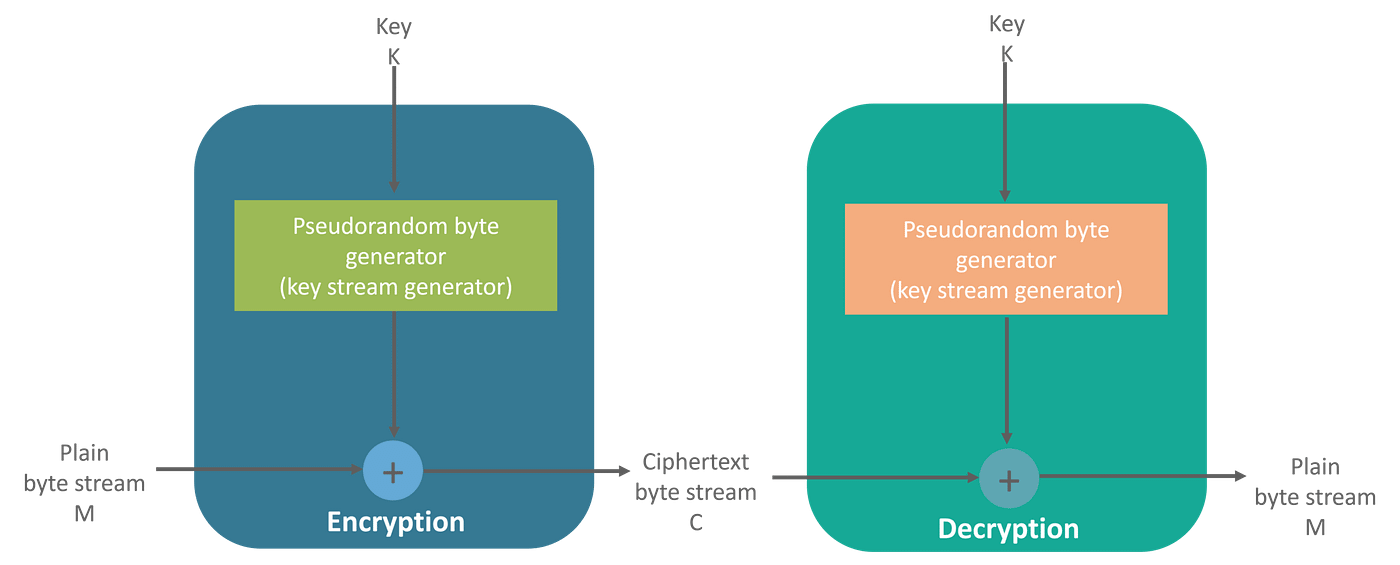## Block Cipher

An encryption method that applies a deterministic algorithm along with a symmetric key to encrypt a block of text, rather than encrypting one bit at a time as in stream ciphers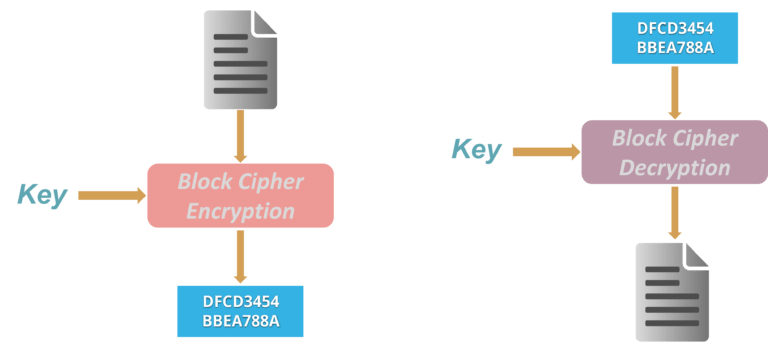Example: A common block cipher, AES, encrypts 128-bit blocks with a key of predetermined length: 128, 192, or 256 bits. Block ciphers are pseudorandom permutation (PRP) families that operate on the fixed size block of bits. PRPs are functions that cannot be differentiated from completely random permutations and thus, are considered reliable until proven unreliable.

# Asymmetric Key Encryption (or Public Key Cryptography)

The encryption process where different keys are used for encrypting and decrypting the information. Keys are different but are mathematically related, such that retrieving the plain text by decrypting ciphertext is feasible.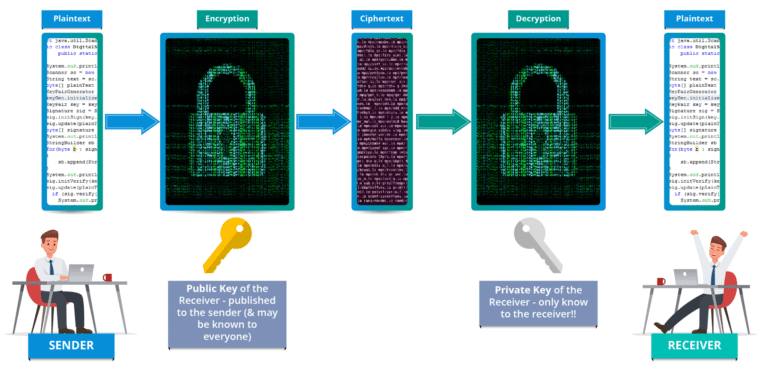RSA is the most widely used form of public key encryption,

## RSA Algorithm

• RSA stands for Rivest, Shamir, and Adelman, inventors of this technique
• Both public and private key are interchangeable
• Variable Key Size (512, 1024, or 2048 bits)

Here’s how keys are generated in RSA algorithm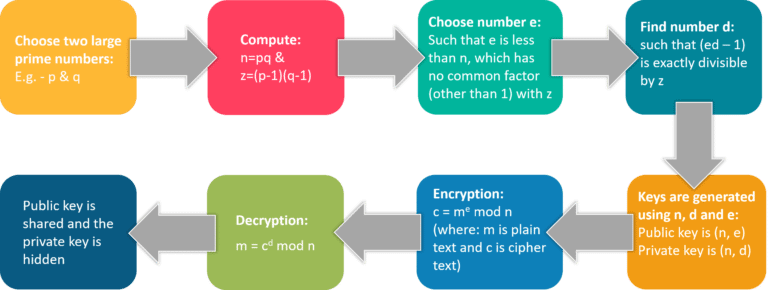## Conclusion

Alright, this was it for “What is Cryptography” article. To safeguard your information and data shared over the internet it is important to use strong encryption algorithms, to avoid any catastrophic situations.

This brings us to the end of our article on What is Cryptography? I hope you found this article informative and added value to your knowledge.